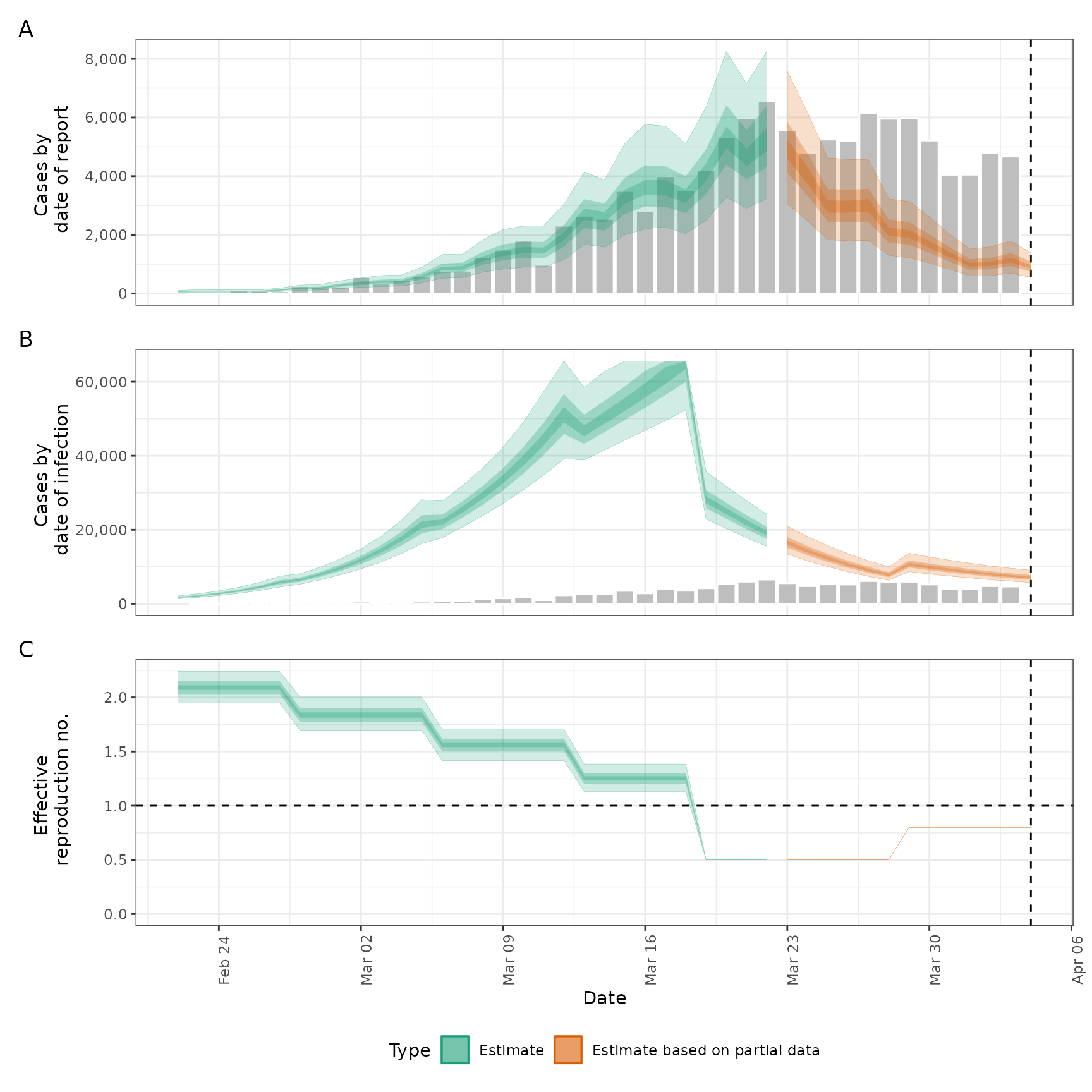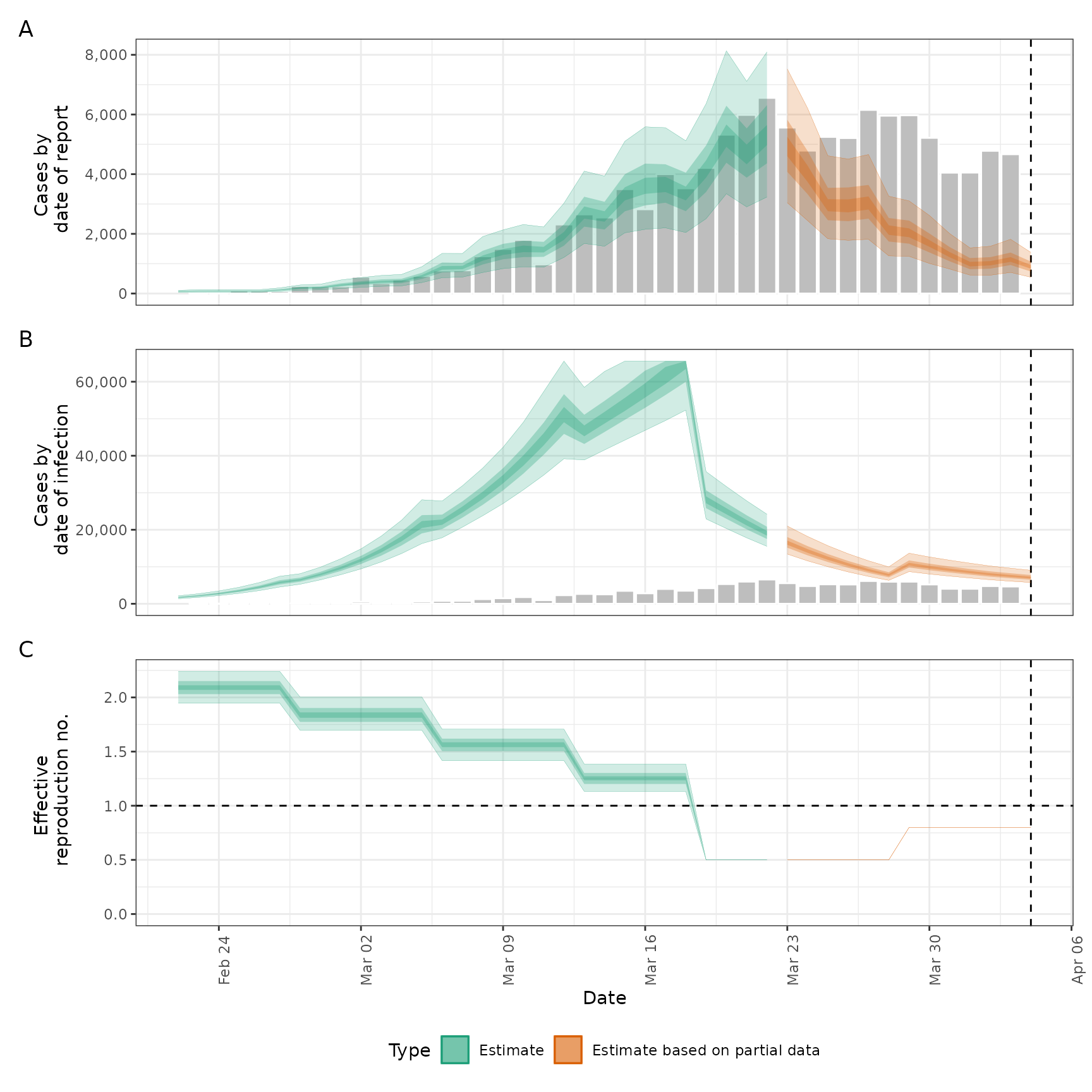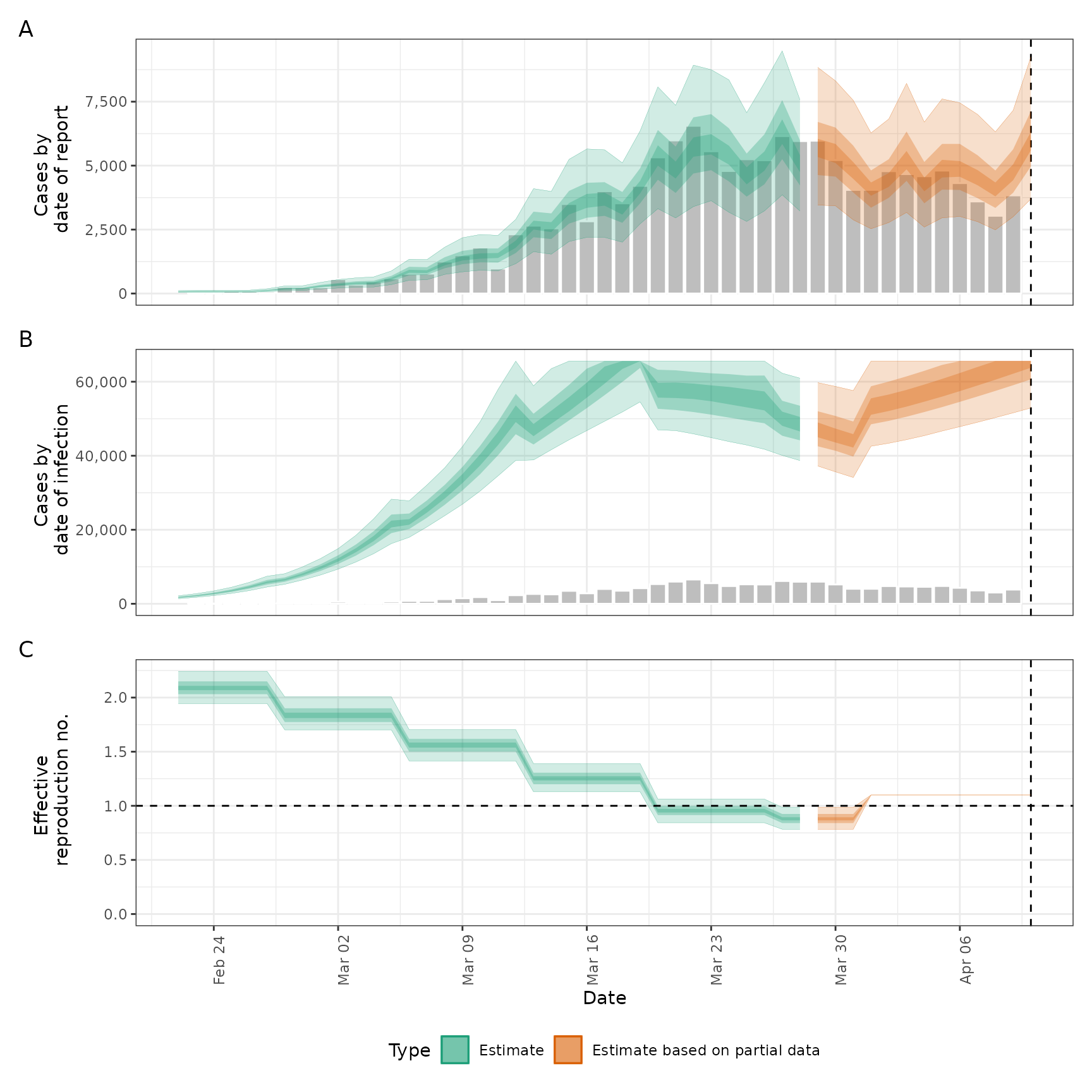This function simulates infections using an existing fit to observed cases but with a modified time-varying reproduction number. This can be used to explore forecast models or past counterfactuals. Simulations can be run in parallel using future::plan.

simulate_infections(
estimates,
R = NULL,
model = NULL,
samples = NULL,
batch_size = 10,
verbose = interactive()
)

## Arguments

estimates

The estimates element of an epinow run that has been done with output = "fit", or the result of estimate_infections with return_fit set to TRUE.

R

A numeric vector of reproduction numbers; these will overwrite the reproduction numbers contained in estimates, except elements set to NA. If it is longer than the time series of reproduction numbers contained in estimates, the values going beyond the length of estimated reproduction numbers are taken as forecast. Alternatively accepts a data.frame containing at least date and value (integer) variables and optionally sample.

model

A compiled stan model as returned by rstan::stan_model.

samples

Numeric, number of posterior samples to simulate from. The default is to use all samples in the estimates input.

batch_size

Numeric, defaults to 10. Size of batches in which to simulate. May decrease run times due to reduced IO costs but this is still being evaluated. If set to NULL then all simulations are done at once.

verbose

Logical defaults to interactive(). Should a progress bar (from progressr) be shown.

## Examples

# \donttest{
# set number of cores to use
options(mc.cores = ifelse(interactive(), 4, 1))
# get example case counts
reported_cases <- example_confirmed[1:50]

# set up example generation time
generation_time <- get_generation_time(disease = "SARS-CoV-2", source = "ganyani")
# set delays between infection and case report
incubation_period <- get_incubation_period(disease = "SARS-CoV-2", source = "lauer")
reporting_delay <- list(
mean = convert_to_logmean(2, 1), mean_sd = 0.1,
sd = convert_to_logsd(2, 1), sd_sd = 0.1, max = 15
)

# fit model to data to recover Rt estimates
est <- estimate_infections(reported_cases,
generation_time = generation_time,
delays = delay_opts(incubation_period, reporting_delay),
rt = rt_opts(prior = list(mean = 2, sd = 0.1)),
gp = gp_opts(
ls_min = 10, boundary_scale = 1.5, ,
basis_prop = 0.1
),
obs = obs_opts(scale = list(mean = 0.1, sd = 0.01))
)

# update Rt trajectory and simulate new infections using it
R <- c(rep(NA_real_, 40), rep(0.5, 10), rep(0.8, 7))
sims <- simulate_infections(est, R)
plot(sims)# with a data.frame input of samples
R_dt <- data.frame(
date = summary(est, type = "parameters", param = "R")\$date,
value = R
)
sims <- simulate_infections(est, R_dt)
plot(sims)#' # with a data.frame input of samples
R_samples <- summary(est, type = "samples", param = "R")
R_samples <- R_samples[, .(date, sample, value)][sample <= 1000][date <= "2020-04-10"]
R_samples <- R_samples[date >= "2020-04-01", value := 1.1]
sims <- simulate_infections(est, R_samples)
plot(sims)# }